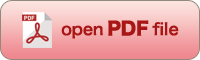[OPTICAL REVIEW Vol. 12, No. 3 (2005) 219-222]
© 2005 The Optical Society of Japan

Paraxial and Aberration Analysis of Off-Axial Optical Systems

Keisuke ARAKI*

Optics Technology Research Center, Canon Inc., 23-10 Kiyohara-Kogyodanchi, Utsunomiya 321-3298, Japan

(Received September 30, 2004; Accepted December 21, 2004)Off-axial optical systems are an extended concept of co-axial optical systems, where deflection surfaces are arranged along the folded reference axis. A newly proposed aberration expression with two kinds of 4-element vectors is simple and comprehensive in its order relation for off-axial optical systems. Using these vectors and tensor algebra, it is shown that aberration expansion coefficients for an arbitrary azimuth can be calculated by transforming the coefficients of 4-element fundamental ray vectors, and the aberration contributions of each surface are in general represented not in simple sum form, but in converted sum form. The aberration contribution of cross terms that exist over the third order, can also be clearly represented using tensor coefficient representation. This paraxial and aberration analysis of off-axial optical systems is very useful in the actual design of optical systems.

Key words: off-axial optical system, folded reference axis, paraxial expansion, paraxial theory, Gaussian matrix, aberration coefficient, cross term, actual lens design

*E-mail: araki.keisuke@canon.co.jp